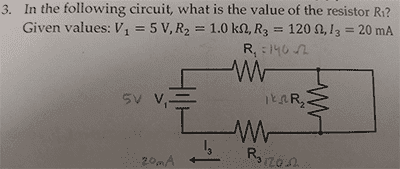# Wire and resistor in parallel

• joel amos

#### joel amos

Member warned about lack of template
If a "normal" looking wire and a resistor are in parallel on a simple circuit diagram, will any current pass through the resistor?

Yes, E/R. It's going to be a very small current since the voltage drop across the wire is going to be very small.

For the purpose of the problem, do you think the current through the resistor would be considered negligible?

You haven't included a problem statement? I've no way of knowing.

You haven't included a problem statement? I've no way of knowing.No difference. You'll want to recalculate R1 it that's actually a "140" I'm reading. As far as the short circuit around R2 for this problem, yes, call it zero.

I'm attempting this by finding the voltage drops at the two known resistors. However, R2 has a voltage drop of .02 A (1,000 Ω) = 20 V. How is this possible, as the battery is only 5 V?

The wire has a nearly zero resistance. It is in parallel with R2. What is the resistance of two resistors in parallel? When one is the next thing to zero?

The wire has a nearly zero resistance.

Ooo. I thought we'd established that the current through the wire was nearly 0.

What is the resistance of two resistors in parallel? When one is the next thing to zero?

I'd say that the resistance would be very small.

very small.
Yes. Sorry, I thought you'd picked up that the wire is carrying nearly all the current, and that the current through the resistor would be just whatever it carries for the very small voltage drop across that length of wire.

I got 130 Ω after solving the following equation for R1:
3.5 V = .02 A (120 Ω + R1)

That's good.

That's good.

Awesome! Thanks for the help!